# Penetration Depth Guide For Biosensor Applications

### Abstract

As visible and infrared waves penetrate human skin, absorption and scattering in the skin layers occurs. The penetration depth of the waves depends on the wavelength and properties of each skin layer. Formulas are presented for generating the absorption and scattering properties as a function of wavelength for each layer of the skin. These properties can then be modeled to determine penetration depth of various wavelengths into human skin. By understanding penetration depth as a function of wavelength, the optimal wavelength can be selected for specific biosensor applications.

### Introduction

Knowledge of the behavior of light as it impinges upon and travels through the skin is crucial for optimizing optical biosensors because it allows for accurate simulation of penetration depth as a function of wavelength. This application note reviews the absorption and reduced scattering coefficients of human skin layers as a function of wavelength. Using these coefficients, penetration depth as a function of wavelength can be simulated and the optimal light source wavelength can be chosen for a given biosensor application.

### Simulating Light Transport Through the Skin

Skin consists of three main layers from the surface: the blood-free epidermis layer (100µm thick), vascularized dermis layer (1mm to 2mm thick), and subcutaneous adipose tissue (from 1mm to 6mm thick, depending on the body site). Typically, the optical properties of these layers are characterized by absorption μa and scattering μs coefficients and the anisotropy factor g. The absorption coefficient characterizes the average number of absorption events per unit path length of photons travelling in the tissue. The main absorbers in the visible spectral range are the blood, hemoglobin, β-carotene, and bilirubin. In the IR spectral range, absorption properties of skin dermis are dominated by absorption of water. The scattering coefficient characterizes the average number of scattering events per unit path length of photons travelling in the tissue, and the anisotropy factor grepresents the average cosine of the scattering angles.

In the following sections, we outline the biological characteristics of each layer and how they affect the propagation and absorption of light.

### Skin Structure and Optical Model

The first and outermost section of human skin is the epidermis. The epidermis can be subdivided into two sublayers: nonliving and living epidermis. Nonliving epidermis or stratum corneum (about 20μm thick) is composed mainly of dead cells, which are highly keratinized with high lipid and protein content. It also has a relatively low water content1. Light absorption in this tissue is low and relatively uniform in the visible region.

The living epidermis (100μm thick) propagates and absorbs light. The absorption properties are mainly determined by a natural chromophore, melanin2. There are two types of melanin: a red/yellow pheomelanin and a brown/black eumelanin, which is associated with skin pigmentation. The melanin absorption level depends on how many melanosomes per unit volume are present. Typically, the volume fraction of the epidermis occupied by melanosomes varies from 1% (lightly pigmented specimens) to 40% (darkly pigmented specimens). The scattering properties of melanin particles are dependent on particle size and can be predicted by the Mie theory.

The dermis is a 0.6mm to 3mm thick structure composed of dense, irregular connective tissue containing nerves and blood vessels. The dermis can be divided into two layers based on the size of the blood vessels3. Smaller vessels lie closer to the skin surface in the papillary dermis while larger blood vessels populate the deeper reticular dermis. Absorption in the dermis is defined by the absorption of hemoglobin, water, and lipids. Since oxyhemoglobin and deoxyhemoglobin have different absorption profiles, the oxygen saturation must be known. For an adult, the arterial oxygen saturation is generally above 95%4. Typical venous oxygen saturation is 60% to 70%5.

The scattering properties of the dermal layers are mainly defined by the fibrous structure of the tissue. Light can scatter on interlaced collagen fibrils and bundles as well as single collagen fibrils. Since the dermal layer is relatively thick compared to the epidermis, the average scattering properties of the skin are dominated by dermal scattering.

The subcutaneous adipose tissue is formed by a collection of fat cells containing stored fat (lipids). Its thickness varies considerably throughout the body: from nonexistent in the eyelids to up to 3cm thick in the abdomen. Absorption of the human adipose tissue is defined by absorption of hemoglobin, lipids and water. The main scatterers of adipose tissue are spherical droplets of lipids, which are uniformly distributed within the fat cells. The diameters of the adipocytes are in the range 15μm to 250μm6 and their mean diameter ranges from 50μm to 120μm7. In the spaces between the cells there are blood capillaries, nerves, and reticular fibrils connecting each cell and providing metabolic activity to the fat tissue.

In this application note, we present a planar five-layer optical model of human skin (see Figure 1) based on the stratified skin layers outlined above. The layers included in the model are the stratum corneum, the living epidermis, the two layers of dermis (papillary and reticular), and the subcutaneous adipose tissue layer. Thickness of the layers as well as typical ranges of blood, water, lipids, and melanin contents, and refractive indices of the layers and mean vessel diameters are presented in Table 1.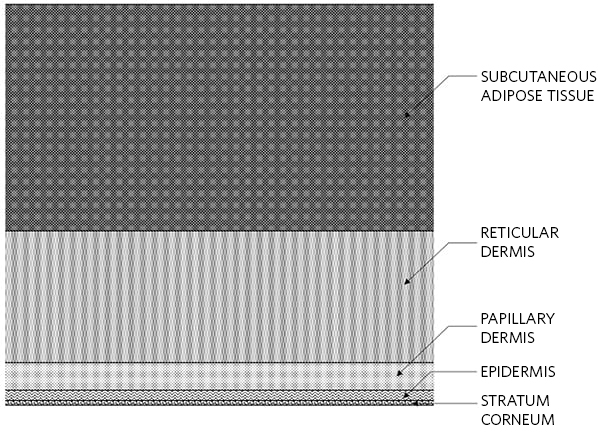Skin layers Thickness (mm) Refractive index Volume fraction of (%) Scattering coefficient at 577nm (bloodless) (cm-1) Mean vessel diameter (µm) Melanosomes Blood Water Lipids Stratum Corneum 0.01 1.55 0.00 0.00 35.00 20.00 300 0 Epidermis 0.1 1.44 1-10 0.00 60.00 15.10 300 0 Papillary Dermis 0.2 1.39 0.00 0.2-4 50.00 17.33 120 6 Reticular Dermis 1.8 1.41 0.00 0.2-5 70.00 17.33 120 15 Subcutaneous Adipose Tissue 3 1.44 0.00 5.00 5.00 75-95 130 75

### Absorption

In the visible and NIR spectral ranges, the absorption coefficient of each layer includes contributions from eumelanin, pheomelanin, oxyhemoglobin, deoxyhemoglobin, bilirubin, ß-carotene, lipids, and water. The spectral extinction coefficients for these pigments, denoted by eu(λ),ph(λ),ohb(λ),dhb(λ),bil(λ), and β(λ), respectively, are given from the curves shown in Figure 2. The total absorption coefficient for the kth layer is given by:

μak(ak,eu(λ))ϑk,mel+(ak,ohb(λ)+ak,dhb(λ)+ak,bil(λ))ϑk,blood

+ (ak,eu(λ))ϑk,water + (ak,lip(λ))ϑk,lip

+ (abase(λ) + ak, β(λ) )(1 - ϑk,mel - ϑk,blood - ϑk,water - ϑ k,lip)

where k = 1,…,5 is the layer number, ϑk,mel, ϑk,blood, ϑk,water, and ϑk,lip are the volume fractions of melanin, blood, water, and lipids in the kth layer, and ak,eu(λ), ak,ph(λ), ak,ohb(λ), ak,dhb(λ), ak,bil(λ),ak,water(λ), ak,lip(λ) and ak,β(λ) are the absorption coefficients of eumelanin, pheomelanin, oxyhemoglobin, deoxyhemoglobin, bilirubin, water, lipids, and β-carotene respectively. abase,β(λ) is the wavelength dependent background tissue absorption coefficient and is given by 7.84e8 × λ -3.255 cm-1.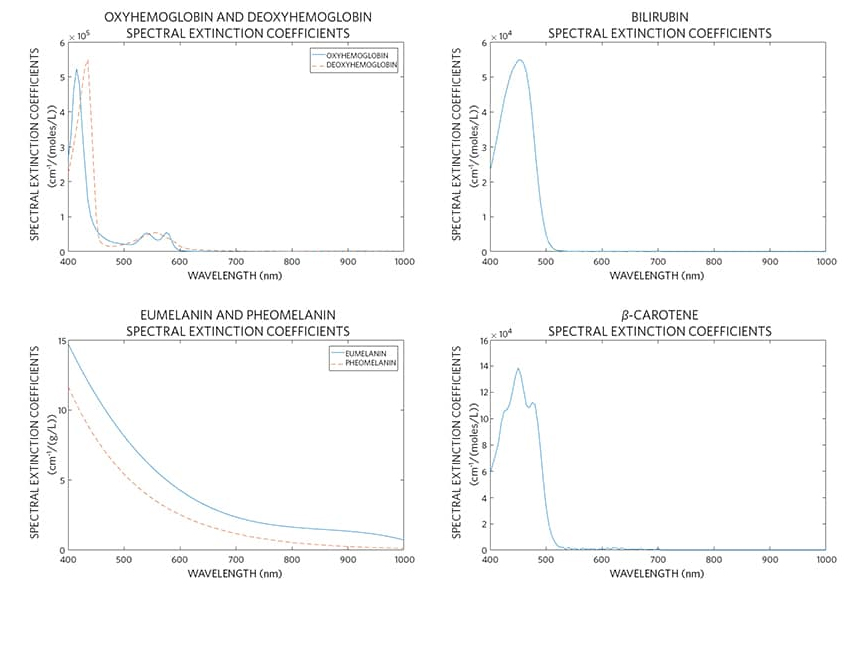The eumelanin and pheomelanin absorption coefficients are given by:

ak.eu(λ) = eu(λ)ck,eu, and ak,ph(λ) = ph(λ)ck,ph

where:

ck,eu = eumelanin concentration (g/L) in the kth layer

ck,ph = pheomelanin concentration (g/L) in the kth layer

The oxyhemoglobin and deoxy hemoglobin absorption coefficients are given by:

ak.ohb(λ) =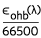ck,hb * γ , and ak,dhb(λ)ck,hb * (1 - γ)

where:

66500 = molecular weight of hemoglobin (g/mol)

ck,hb = hemoglobin concentration of the blood (g/L) in the kth layer

γ = ratio of oxyhemoglobin to the total hemoglobin concentration.

The absorption coefficient of bilirubin is given by:

ak,bil(λ) =ck,bil

where:

585 = molecular weight of bilirubin (g/mol)

ck,bil = bilirubin concentration (g/L) in the kth layer

The β-carotene absorption coefficient ak,β(λ) is given by:

ak,β(λ) =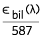ck,β

where:

537 = molecular weight of β-carotene (g/mol)

ck,β = β-carotene concentration (g/L) in the kth layer

The absorption coefficient of water is given by:

ak,water(λ) = water(λ)ck,water3

where:

ck,water = water concentration (g/L) in the kth layer.

The lipid absorption coefficient is given by:

ak,lip(λ) = water(λ)ck,lip3

where:

ck,lip = lipid concentration (g/L) in the kth layer.

ak,lip(λ) = water(λ)ck,lip3

### Scattering

The total scattering coefficient for the kth layer can be defined as:

μs(λ) = ϑk,bloodCkμsblood(λ)+(1-ϑk,blood)μsTk(λ).

where Ck is a correction factor dependent on the mean vessel diameter and the blood scattering coefficient as a function of wavelength and μsTk is the total scattering coefficient of the bloodless tissue layer.

The following relations can be used for Ck8:

Ck =where dk,vesselsis the blood vessels diameter (cm) in the kth layer. In the case of collimated illumination of the vessels the coefficients a and b have values a = 1.007 and b = 1.228. In the case of diffuse illumination of the vessels the coefficients a and b have values a = 1.482 and b = 1.151.

The total scattering coefficient of the bloodless tissue is given by9:

μsTk(λ) = μs0k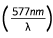,

where μs0k are the scattering coefficients at the reference wavelength 577nm listed in Table 1. Note: μsTk, falls monotonically with the increase in the wavelength.

The expression for the anisotropy of scattering may be constructed to include the contribution from blood9:

gk (λ) =,

where gk (λ) is the anisotropy factor of the bloodless tissue and

gTk (λ) = 0.7645 + 0.2355 [1 - exp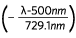].

Finally, the reduced scattering coefficients is defined as μs'k(λ) = μsk(λ)(1 - gk(λ)).

### H2Optical Model and Computer Simulations

To determine penetration depth as a function of wavelength, Zemax OpticStudio® software was utilized. The software uses a Monte Carlo (MC) method to trace optical rays propagating in complex inhomogeneous, randomly scattering and absorbing media. Basic MC modeling of an individual photon packets trajectory consists of the sequence of the elementary simulations: photon path length generation, scattering and absorption events, reflection and/or refraction on the medium boundaries. The specular reflection from the air-tissue surface is also considered in the simulations. At the scattering site, a new photon packet direction is determined according to the Henyey-Greenstein scattering phase function:

fHG(Θ) =where Θ is the polar scattering angle. The distribution over the azimuthal scattering angle was assumed as uniform. MC technique requires values of absorption and scattering coefficients and anisotropy factor of each skin layer, its thickness and refractive index. Additionally, the mean path defined as the inverse of the scattering coefficient is required.

### Results and Discussion

Using optical properties presented above, Henyey-Greenstein scattering phase function, and Zemax OpticStudio, we can simulate any biosensor configuration and determine the maximum penetration depth as a function of wavelength. For example, we have taken the following typical LED-PD biosensor configuration (Table 2 and Figure 3) and skin properties shown in Table 3, and simulated the maximum penetration depth as a function of wavelength.

 Configuration property Value Unit Distance between LED and PD 3.5 mm PD size 0.4 x 0.4 mm x mm LED radiation pattern Lambertian - LED divergence angle FWHM 60 degrees LED size 340 x 340 µm x m LED/PD top to Stratum Corneum spacing 0.5 mmSkin layers Volume fraction of (%) Concentration of (g/L) Melanosomes Blood Water Lipids Beta carotene Eumelanin Pheomelanin Bilirubin Hemoglobin in blood Stratum Corneum 0.00 0.00 35.00 20.00 2.10E–04 0 0 0 0 Epidermis 1-10 0.00 60.00 15.10 2.10E–04 80 12 0 0 Papillary Dermis 0.00 0.2-4 50.00 17.33 7.00E–05 0 0 0.05 150 Reticular Dermis 0.00 0.2-5 70.00 17.33 7.00E–05 0 0 0.05 150 Subcutaneous Adipose Tissue 0.00 5.00 5.00 75-95 0 0 0 0.05 150

The absorption coefficients of the skin layers have been calculated in accordance with the presented optical model and are shown in Figure 4.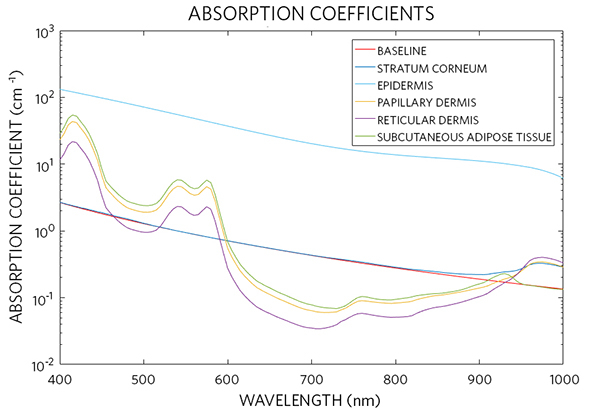The scattering coefficient, anisotropy factor, and mean path of the skin layers have been calculated in accordance with the presented model, and the result presented in Figure 5, Figure 6, and Figure 7.The penetration depth of light into a biological tissue is an important parameter used to determine the performance of a biosensor. Penetration depth is the distance into the skin that the light has traveled. Simulation of the optical penetration depth has been performed with the absorption and reduced scattering coefficient values presented above. Simulation results are shown in Figure 8.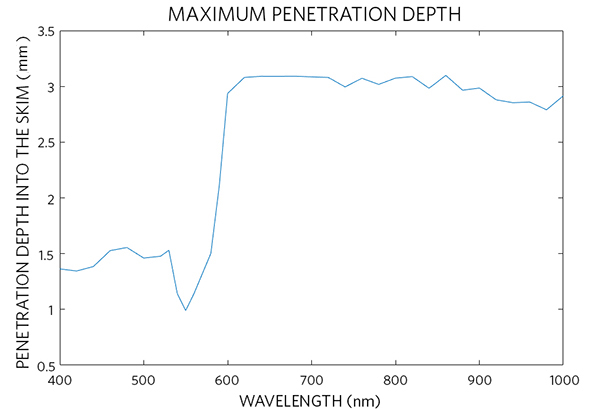### Conclusion

In this article, we have modeled human skin tissue with a five-layer structure where each layer represents its corresponding anatomical layer. To simulate light tissue interaction, the biological characteristics of each layer is moded with three wavelength-dependent numbers, absorption coefficient, scattering coefficient, and anisotropy factor. Then, we have used a commercial ray tracing software to calculate penetration depth of light into skin tissue for simulating the performance of optical biosensor architectures.

### References

1. K. S. Stenn, "The skin, " Cell and Tissue Biology ed. by L. Weiss, Baltimore: Urban & Shwarzenberg, 541-572 (1988).
2. M. R. Chedekel, "Photophysics and Photochemistry of Melanin, " In: L. Zeise, M. R. Chedekel and T. B. Fitzpatrick, Eds., Melanin: Its Role in Human Photoprotection, Valdenmar, Overland Park, pp. 11-22 (1994).
3. T. J. Ryan, "Cutaneous Circulation," in Physiology, Biochemistry, and Molecular Biology of the Skin, 1, ed. by L.A. Goldsmith, Oxford University Press, Oxford (1991).
4. A. Zourabian, A. Siegel, B, Chance, N. Ramanujan, M. Rode and D. A. Boas, "Trans-abdominal monitoring of fetal arterial blood oxygenation using pulse oximetry, " J. Biomed. Opt. 5 391–405 (2000).
5. T. Hamaoka et al, "Quantification of ischemic muscle deoxygenation by near infrared time-resolved spectroscopy, " J. Biomed. Opt. 5 102–5 (2000).
6. M. I. Gurr, R. T. Jung, M. P. Robinson and W. P. T. James, "Adipose tissue cellularity in man: the relationship between fat cell size and number, the mass and distribution of body fat and the history of weight gain and loss," Int. J. Obesity 6 419–36 (1982).
7. Y. Taton "Obesity, Pathphysiology, Diagnostics, Therapy", Warsaw: Medical Press (1981).
8. W. Verkruysse, G. W. Lucassen, J. F. de Boer, D. J. Smithies, J. S. Nelson, M. J. C. van Gemert, "Modelling light distributions of homogeneous versus discrete absorbers in light irradiated turbid media," Phys. Med. Biol. 42, 51-65 (1997).
9. G. Altshuler, M. Smirnov, I. Yaroslavsky, "Lattice of optical islets: a novel treatment modality in photomedicine," Journal of Physics D: Applied Physics 38, 2732-2747 (2005).

OpticStudio is a registered trademark of Zemax, LLC.